# R语言简单绘图（二）

1、条形图—barplot()

barplot(c(1,2,4,2,6,4,3,5))
barplot(c(1,2,4,2,6,4,3,5), horiz = TRUE)

counts = table(Arthritis$Improved) barplot(counts, horiz = TRUE) counts = table(Arthritis$Improved, Arthritis$Treatment) barplot(counts, beside = TRUE) state <- data.frame(state.region, state.x77) x <- aggregate(state$Illiteracy, by=list(state.region), FUN=mean)
barplot(x$x, names.arg =x$Group.1)

par(mar=c(5,8,4,2))
counts <- table(Arthritis$Improved) barplot(counts, horiz = TRUE, cex.names = 0.8, names.arg = c("no improved", "some", "marked")) par(las=2) barplot(counts, horiz = TRUE, cex.names = 0.8, names.arg = c("no improved", "some", "marked"))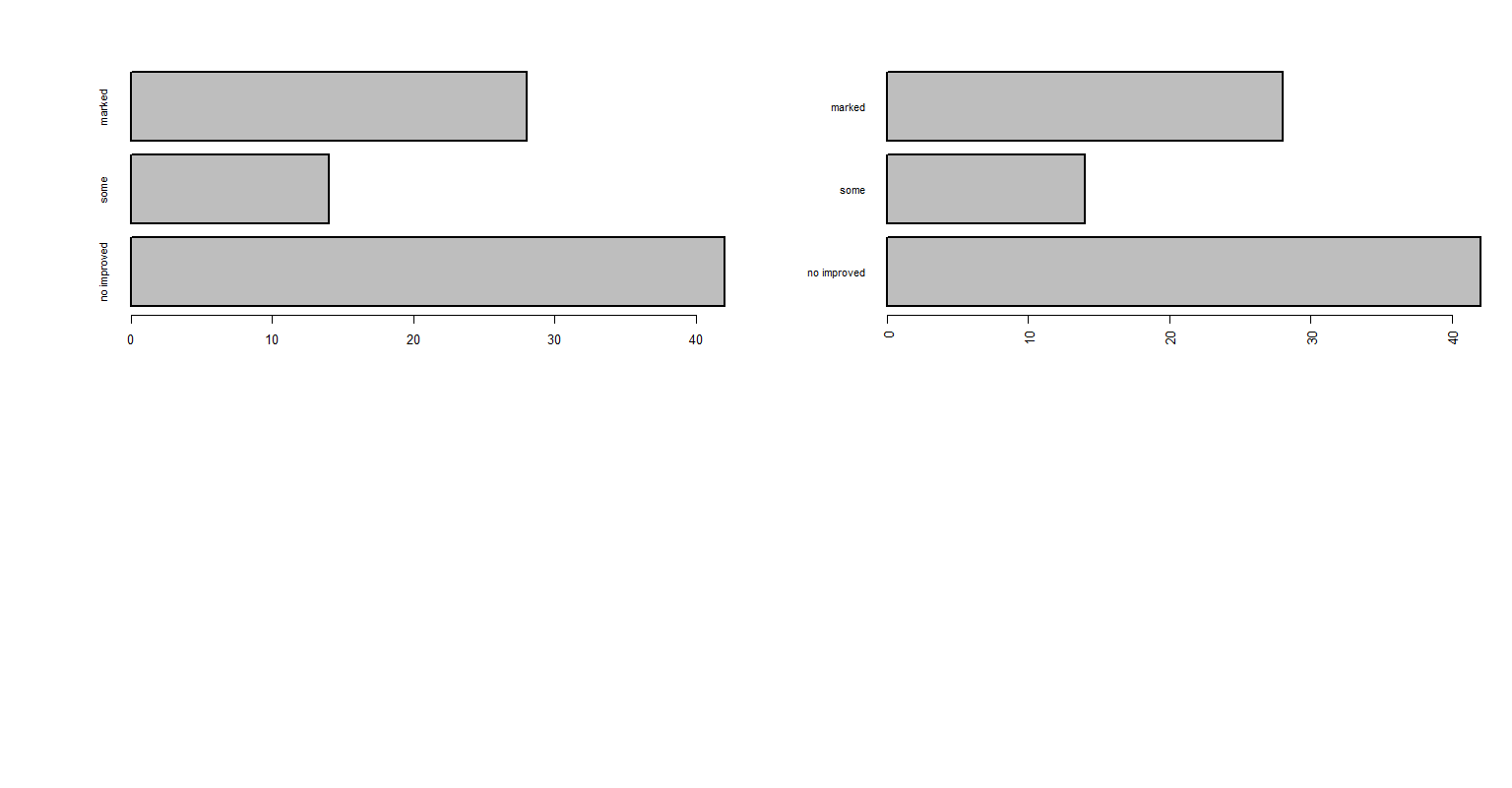2、饼图—pie() par(mfrow=c(2,2)) x <- c(10,12, 4,16, 8) lab <- c("US", "UK", "Australia", "Germany", "France") pie(x, lab, main="Simple Pie Chart") pct <- round(x/sum(x)*100) lab1 <- paste(lab, " ", pct, "%", sep = " ") pie(x,lab1, col = rainbow(length(lab1)), main = "Pie Chart with Percentage") pie3D(x, explode=0.1, main="3D Pie Chart") fan.plot(x, labels = lab, main="Fan Plot") # 扇形图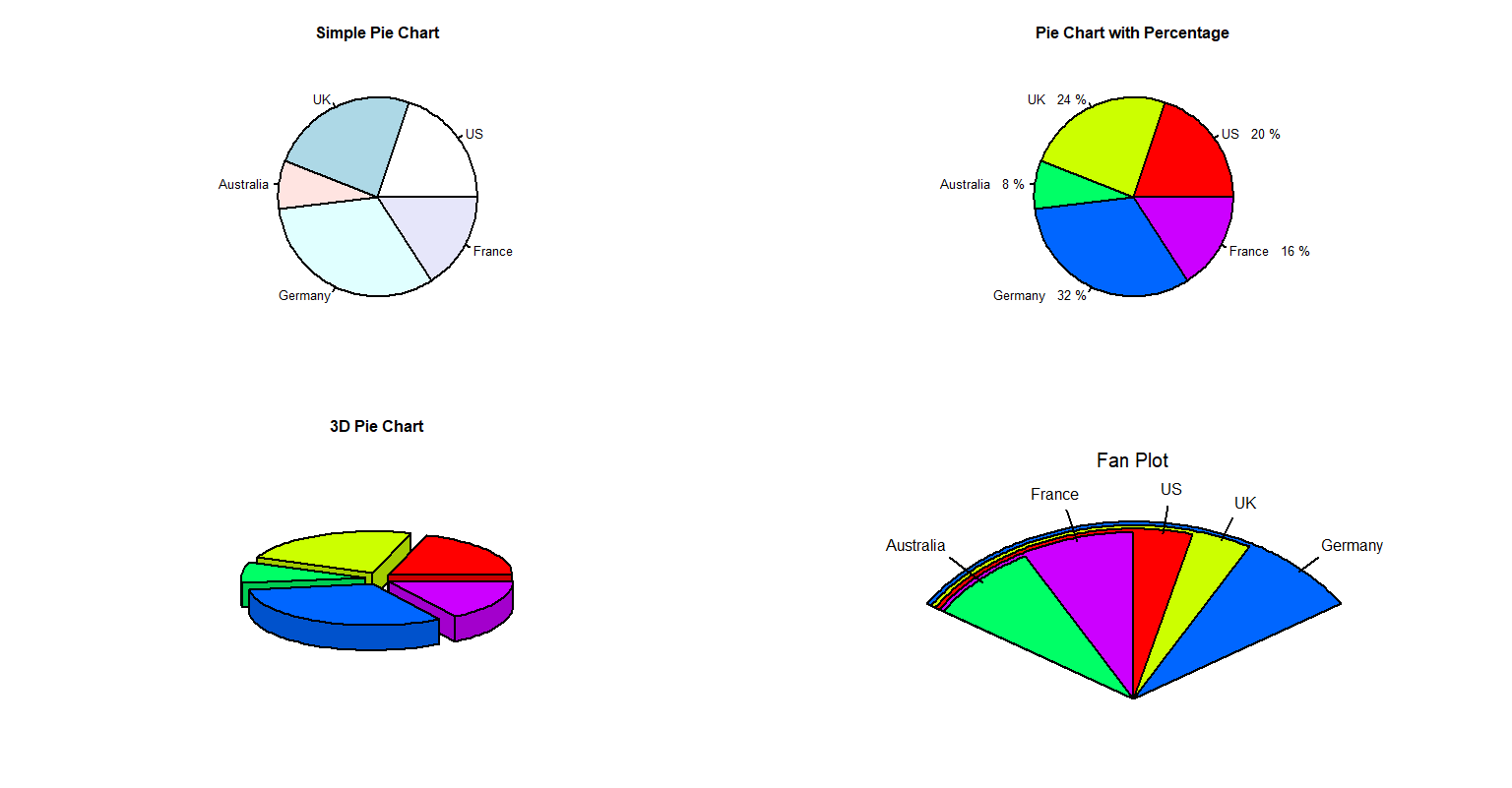3、直方图—hist() attach(mtcars) # 导入数据 x <- mtcars$mpg
hist(x)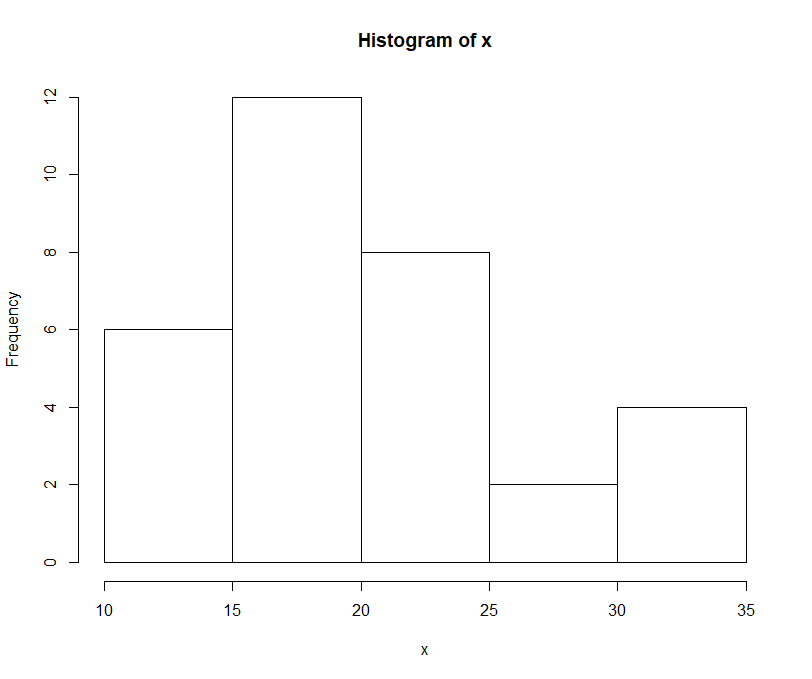hist(x,breaks = 12, col="red",xlab = "Miles Per Callon")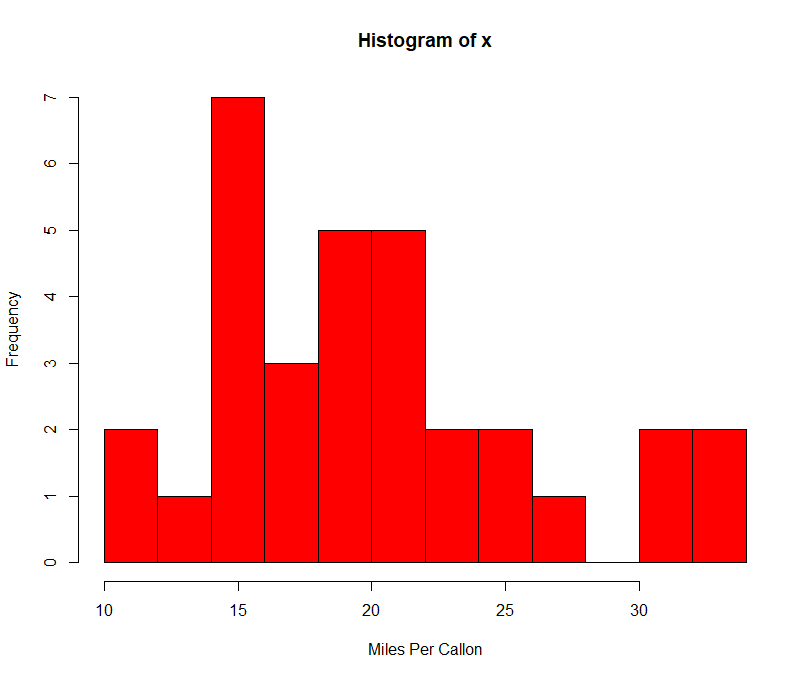hist(x, freq = FALSE, breaks = 12, col = "green",xlab = "Miles Per Callon")
lines(density(x), col="red", lwd=2)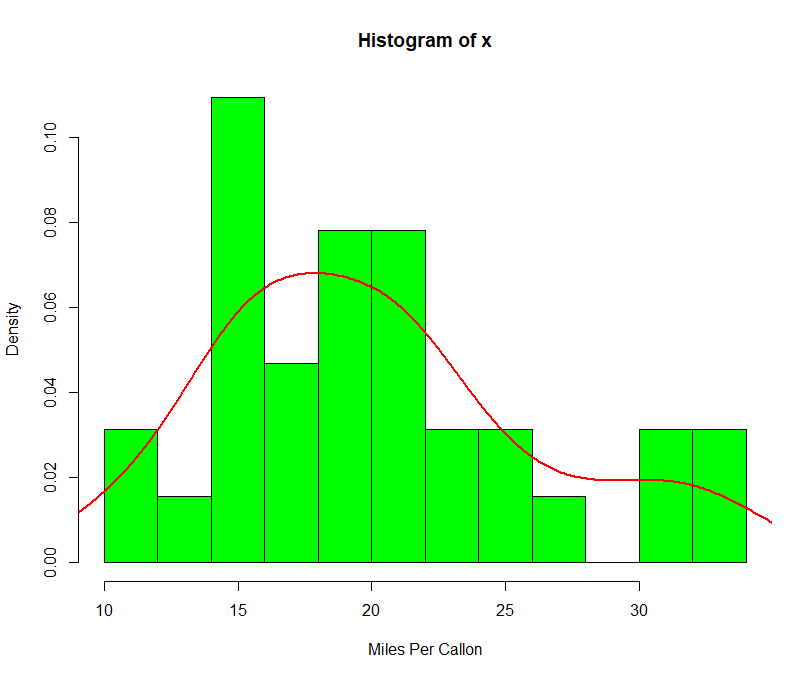4、箱线图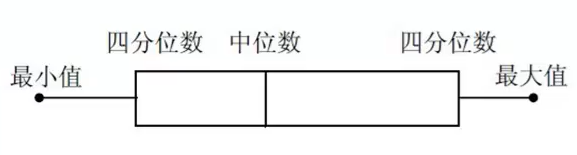boxplot(mtcars\$mpg, mian="Box plot", ylab="Mile per gallon")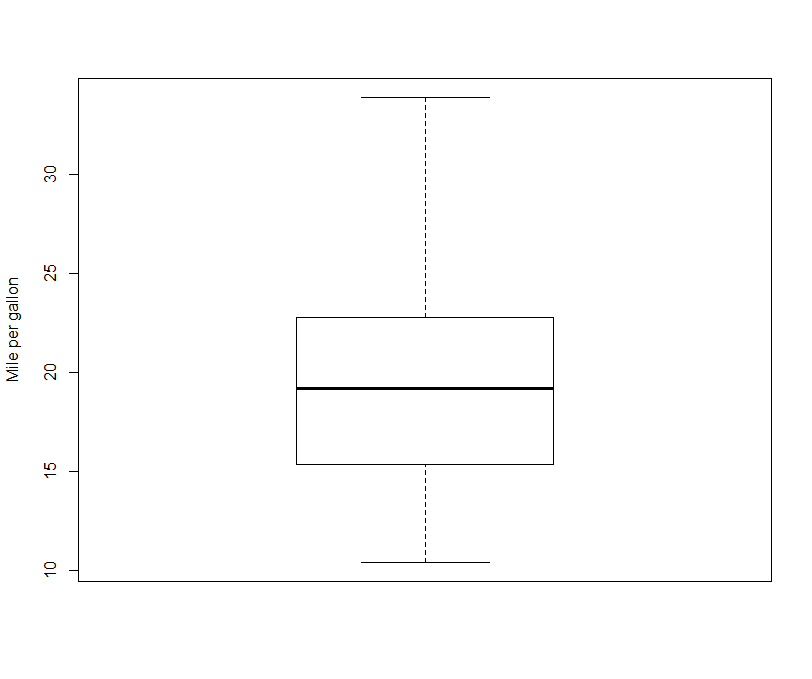boxplot(mpg~cyl, data=mtcars, main="Car mileage data", xlab="Number of cylinders", ylab="Miles per gallon") # 对比图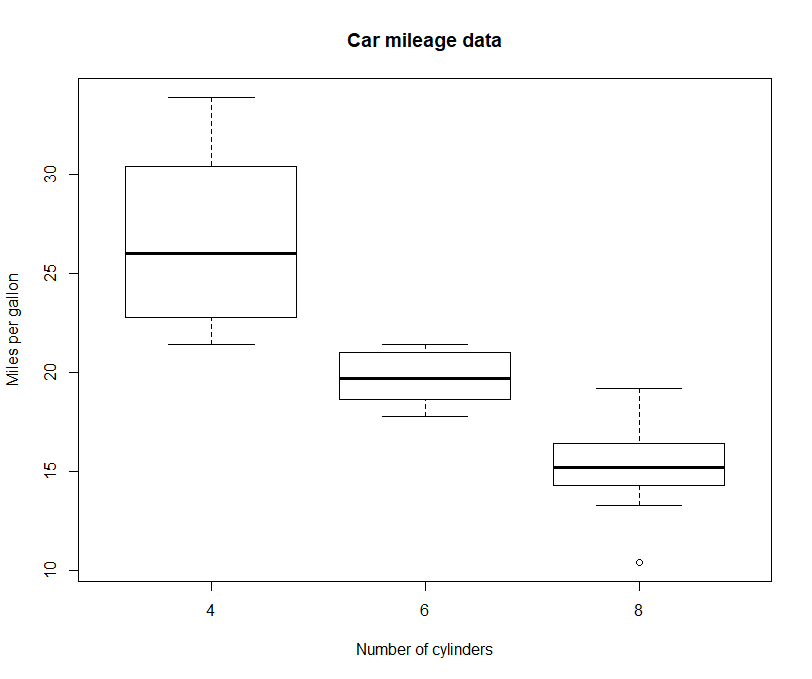07-26
06-251万+02-251万+
04-07111
05-073716
11-294万+
10-062万+
02-141597
01-084万+
05-252万+